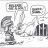# How to script drawing horizontal lines on every 50 cents number?

S

#### scriptorswim

##### New member
Pretty much the question folks. I have seen some codes from google for creating horizontal lines for the current price/position (in which they didnt work really) but i tried to mess with them to create this but been unsuccessful. Basically, if we have SPY on our chart, i want to see hortizontal lines at 307,307.5,308,308.5 that are close to the current price (50 instances?) for today, anyone have the script or interested in mocking something up?

Last edited:#### BenTen

Staff
VIP
Something like this would work

Code:
``````input level = 100;

plot a = level + 5;

plot b = level + 10;``````

L

#### Len20

##### Member
I'm working on something similar which draws a horizontal line when price goes near an even number relative to the price of the stock.

I modified it to plot the nearest 50 cents above and below the current price, so it adjusts with the current price. It's just two horizontal lines, but I figured it might be useful anyways.

Code:
``````# Even Number Lines by Len20

input step = .50;

def price = close;
def upper = roundUp(price / step , 0) * step;
def lower = roundDown(price/ step , 0) * step;
def upperLine = if !isNaN(price) and isNaN(price[-1]) then upper else if isNan(price) then upperLine else double.NaN;
def lowerLine = if !isNaN(price) and isNaN(price[-1]) then lower else if isNan(price) then lowerLine else double.NaN;

plot above1 = upperLine;
plot below1 = lowerLine;``````Script Function and Line Drawing Logic for ThinkorSwim by JQ Tutorials 2